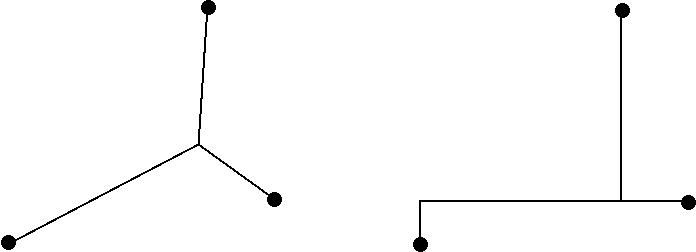# Steiner Tree Problem InstancesThis page contains various Steiner tree problem instances, together with optimal objective values (or best known bounds):

• MPC Paper: All of the instances used in our paper: Juhl, Warme, Winter and Zachariasen: The GeoSteiner Software Package for Computing Steiner Trees in the Plane: An Updated Computational Study. Mathematical Programming Computation 10, 487-532 (2018).

• EFST: These instances, made available for the challenge by Daniel Juhl, David M. Warme, Pawel Winter, and Martin Zachariasen, are obtained by full Steiner tree generation for the classical Euclidean Steiner tree problem in the plane (as explained in their challenge presentation). By solving the graph problem, the underlying Euclidean Steiner tree problem is solved to optimality (modulo rounding errors in the transformation).

Each instance has one version with fractional numbers suitable for 64-bit floating point types, and another where the weights are suitable for 64-bit unsigned integer types. In the latter case the original distances have been scaled with a suitable factor of 10^k and rounded to the nearest integer such that the sum of edge weights is less than 2^64; these instance sets have suffix "INT". The authors recommend using the floating point instances if at all possible, since scaling and rounding to integers in general results in a worse approximation of the original Euclidean distances.

There are four sets of instances:

The best known bounds for these instances as of March 24, 2015 are available as a text file. For instances in which optimality is not proven, a lower bound is also provided. (INT instances last updated on May 10, 2015.)

http://www.geosteiner.com/

webmaster@geosteiner.com, June 25, 2022